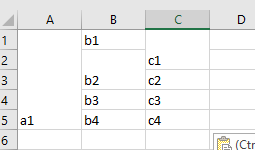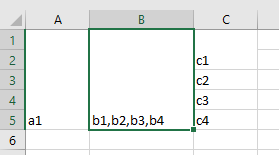# [Solved] Merging Rows of column B with the count of already merged rows A

1. Merging cells in a spreadsheet means taking two or more cells and
constructing a single cell out of them. When you merge two or more
adjacent horizontal or vertical cells, the cells become one larger
cell that is displayed across multiple columns or rows. When you
merge multiple cells, the contents of only one cell (the upper-left
cell for left-to-right languages, or the upper-right cell for
right-to-left languages) appear in the merged cell. The contents of
the other cells that you merge are deleted. For more details please
go through this MSDN article Merge and unmerge
cells

Simple VBA code for Merging Cell

``````Sub merg_exp_1()
ActiveSheet.Range("A1:C10").Merge
End Sub
``````
1. Now let us see, If we merge a row what happens. Sample code for this
exercise though general is being tested for one situation only and

``````Sub Merge_Rows()
Dim rng As Range
Dim rrow As Range
Dim rCL As Range
Dim out As String
Dim dlmt As String
dlmt = ","
Set rng = ActiveSheet.Range("A1:C5")
For Each rrow In rng.Rows
out = ""
For Each rCL In rrow.Cells
If rCL.Value <> "" Then
out = out & rCL.Value & dlmt
End If
Next rCL
rrow.Merge
If Len(rrow.Cells(1).Value) > 0 Then
rrow.Cells(1).Value = Left(out, Len(out) - 1)
End If
Next rrow
End Sub
``````

Sample data before and after running the program is shown. You can see this won’t meet your objective.

1. Next we can try merging by column approach. Here also we are trying
for one column i.e. Column B to see the effect. Sample code as
follows.

``````Sub Merge_col_exp()

Dim cnum As Integer
Dim rng As Range
Dim str As String

For i = ActiveSheet.UsedRange.Rows.Count To 1 Step -1
cnum = Cells(i, 1).MergeArea.Count
Set rng = Range(Cells(i, 2), Cells(i - cnum + 1, 2)) ' only to demonstrate working in 2nd column

For Each cl In rng
If Not IsEmpty(cl) Then str = str + "," + cl
Next
If str <> "" Then str = Right(str, Len(str) - 1)

rng.Merge
rng = str